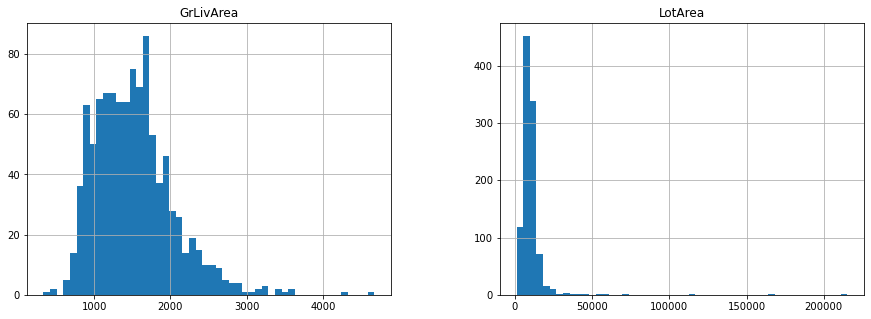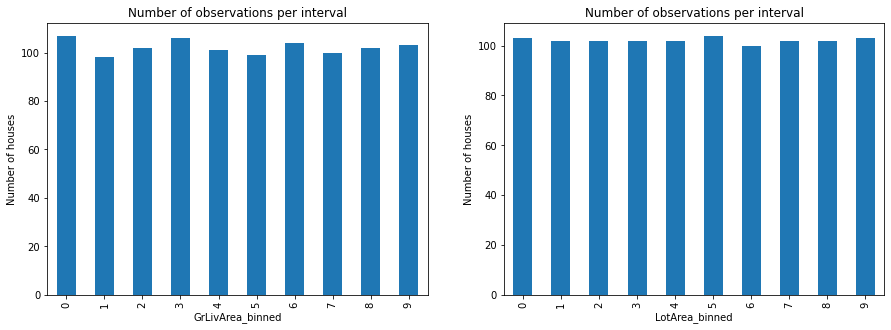# EqualFrequencyDiscretiser¶

The EqualFrequencyDiscretiser() divides continuous numerical variables into contiguous equal frequency intervals, that is, intervals that contain approximately the same proportion of observations.

The interval limits are determined by the quantiles. The number of intervals, i.e., the number of quantiles in which the variable should be divided is determined by the user.

Note

For this demonstration, we use the Ames House Prices dataset produced by Professor Dean De Cock:

Dean De Cock (2011) Ames, Iowa: Alternative to the Boston Housing Data as an End of Semester Regression Project, Journal of Statistics Education, Vol.19, No. 3

http://jse.amstat.org/v19n3/decock.pdf

https://www.tandfonline.com/doi/abs/10.1080/10691898.2011.11889627

The version of the dataset used in this notebook can be obtained from Kaggle

In :
import pandas as pd
import numpy as np
import matplotlib.pyplot as plt

from sklearn.model_selection import train_test_split

from feature_engine.discretisation import EqualFrequencyDiscretiser

plt.rcParams["figure.figsize"] = [15,5]

In :
data = pd.read_csv('housing.csv')


Out:
Id MSSubClass MSZoning LotFrontage LotArea Street Alley LotShape LandContour Utilities ... PoolArea PoolQC Fence MiscFeature MiscVal MoSold YrSold SaleType SaleCondition SalePrice
0 1 60 RL 65.0 8450 Pave NaN Reg Lvl AllPub ... 0 NaN NaN NaN 0 2 2008 WD Normal 208500
1 2 20 RL 80.0 9600 Pave NaN Reg Lvl AllPub ... 0 NaN NaN NaN 0 5 2007 WD Normal 181500
2 3 60 RL 68.0 11250 Pave NaN IR1 Lvl AllPub ... 0 NaN NaN NaN 0 9 2008 WD Normal 223500
3 4 70 RL 60.0 9550 Pave NaN IR1 Lvl AllPub ... 0 NaN NaN NaN 0 2 2006 WD Abnorml 140000
4 5 60 RL 84.0 14260 Pave NaN IR1 Lvl AllPub ... 0 NaN NaN NaN 0 12 2008 WD Normal 250000

5 rows × 81 columns

In :
# let's separate into training and testing set
X = data.drop(["Id", "SalePrice"], axis=1)
y = data.SalePrice

X_train, X_test, y_train, y_test = train_test_split(
X, y, test_size=0.3, random_state=0)

print("X_train :", X_train.shape)
print("X_test :", X_test.shape)

X_train : (1022, 79)
X_test : (438, 79)

In :
# we will use two continuous variables for transformation

X_train[["LotArea", 'GrLivArea']].hist(bins=50)

plt.show()The EqualFrequencyDiscretiser() works only with numerical variables. A list of variables can be passed as argument. Alternatively, the discretiser will automatically select and transform all numerical variables.

The EqualFrequencyDiscretiser() first finds the boundaries for the intervals or quantiles for each variable, fit.

Then it transforms the variables, that is, it sorts the values into the intervals, transform.

In :
'''
Parameters
----------

q : int, default=10
Desired number of equal frequency intervals / bins. In other words the
number of quantiles in which the variables should be divided.

variables : list
The list of numerical variables that will be discretised. If None, the
EqualFrequencyDiscretiser() will select all numerical variables.

return_object : bool, default=False
Whether the numbers in the discrete variable should be returned as
numeric or as object. The decision is made by the user based on
whether they would like to proceed the engineering of the variable as
if it was numerical or categorical.

return_boundaries: bool, default=False
whether the output should be the interval boundaries. If True, it returns
the interval boundaries. If False, it returns integers.
'''

efd = EqualFrequencyDiscretiser(q=10, variables=['LotArea', 'GrLivArea'])

efd.fit(X_train)

Out:
EqualFrequencyDiscretiser(q=10, return_boundaries=False, return_object=False,
variables=['LotArea', 'GrLivArea'])
In :
# binner_dict contains the boundaries of the different bins
efd.binner_dict_

Out:
{'LotArea': [-inf,
5007.1,
7164.6,
8165.700000000001,
8882.0,
9536.0,
10200.0,
11046.300000000001,
12166.400000000001,
14373.9,
inf],
'GrLivArea': [-inf,
912.0,
1069.6000000000001,
1211.3000000000002,
1344.0,
1479.0,
1603.2000000000003,
1716.0,
1893.0000000000005,
2166.3999999999996,
inf]}
In :
train_t = efd.transform(X_train)
test_t = efd.transform(X_test)

In :
# the numbers are the different bins into which the observations
# were sorted
train_t['GrLivArea'].unique()

Out:
array([8, 3, 0, 4, 1, 2, 6, 9, 7, 5], dtype=int64)
In :
# the numbers are the different bins into which the observations
# were sorted
train_t['LotArea'].unique()

Out:
array([4, 0, 2, 3, 1, 7, 5, 8, 6, 9], dtype=int64)
In :
# here I put side by side the original variable and the transformed variable
tmp = pd.concat([X_train[["LotArea", 'GrLivArea']], train_t[["LotArea", 'GrLivArea']]], axis=1)
tmp.columns = ["LotArea", 'GrLivArea',"LotArea_binned", 'GrLivArea_binned']

Out:
LotArea GrLivArea LotArea_binned GrLivArea_binned
64 9375 2034 4 8
682 2887 1291 0 3
960 7207 858 2 0
1384 9060 1258 4 3
1100 8400 438 3 0
In :
# in  equal frequency discretisation, we obtain the same amount of observations
# in each one of the bins.
plt.subplot(1,2,1)
tmp.groupby('GrLivArea_binned')['GrLivArea'].count().plot.bar()
plt.ylabel('Number of houses')
plt.title('Number of observations per interval')

plt.subplot(1,2,2)
tmp.groupby('LotArea_binned')['LotArea'].count().plot.bar()
plt.ylabel('Number of houses')
plt.title('Number of observations per interval')

plt.show()### Return interval limits instead¶

In :
# Now, let's return bin boundaries instead

efd = EqualFrequencyDiscretiser(
q=10, variables=['LotArea', 'GrLivArea'], return_boundaries=True)

efd.fit(X_train)

Out:
EqualFrequencyDiscretiser(q=10, return_boundaries=True, return_object=False,
variables=['LotArea', 'GrLivArea'])
In :
train_t = efd.transform(X_train)
test_t = efd.transform(X_test)

In :
# the numbers are the different bins into which the observations
# were sorted
np.sort(np.ravel(train_t['GrLivArea'].unique()))

Out:
array([Interval(-inf, 912.0, closed='right'),
Interval(912.0, 1069.6, closed='right'),
Interval(1069.6, 1211.3, closed='right'),
Interval(1211.3, 1344.0, closed='right'),
Interval(1344.0, 1479.0, closed='right'),
Interval(1479.0, 1603.2, closed='right'),
Interval(1603.2, 1716.0, closed='right'),
Interval(1716.0, 1893.0, closed='right'),
Interval(1893.0, 2166.4, closed='right'),
Interval(2166.4, inf, closed='right')], dtype=object)
In :
np.sort(np.ravel(test_t['GrLivArea'].unique()))

Out:
array([Interval(-inf, 912.0, closed='right'),
Interval(912.0, 1069.6, closed='right'),
Interval(1069.6, 1211.3, closed='right'),
Interval(1211.3, 1344.0, closed='right'),
Interval(1344.0, 1479.0, closed='right'),
Interval(1479.0, 1603.2, closed='right'),
Interval(1603.2, 1716.0, closed='right'),
Interval(1716.0, 1893.0, closed='right'),
Interval(1893.0, 2166.4, closed='right'),
Interval(2166.4, inf, closed='right')], dtype=object)
In [ ]: